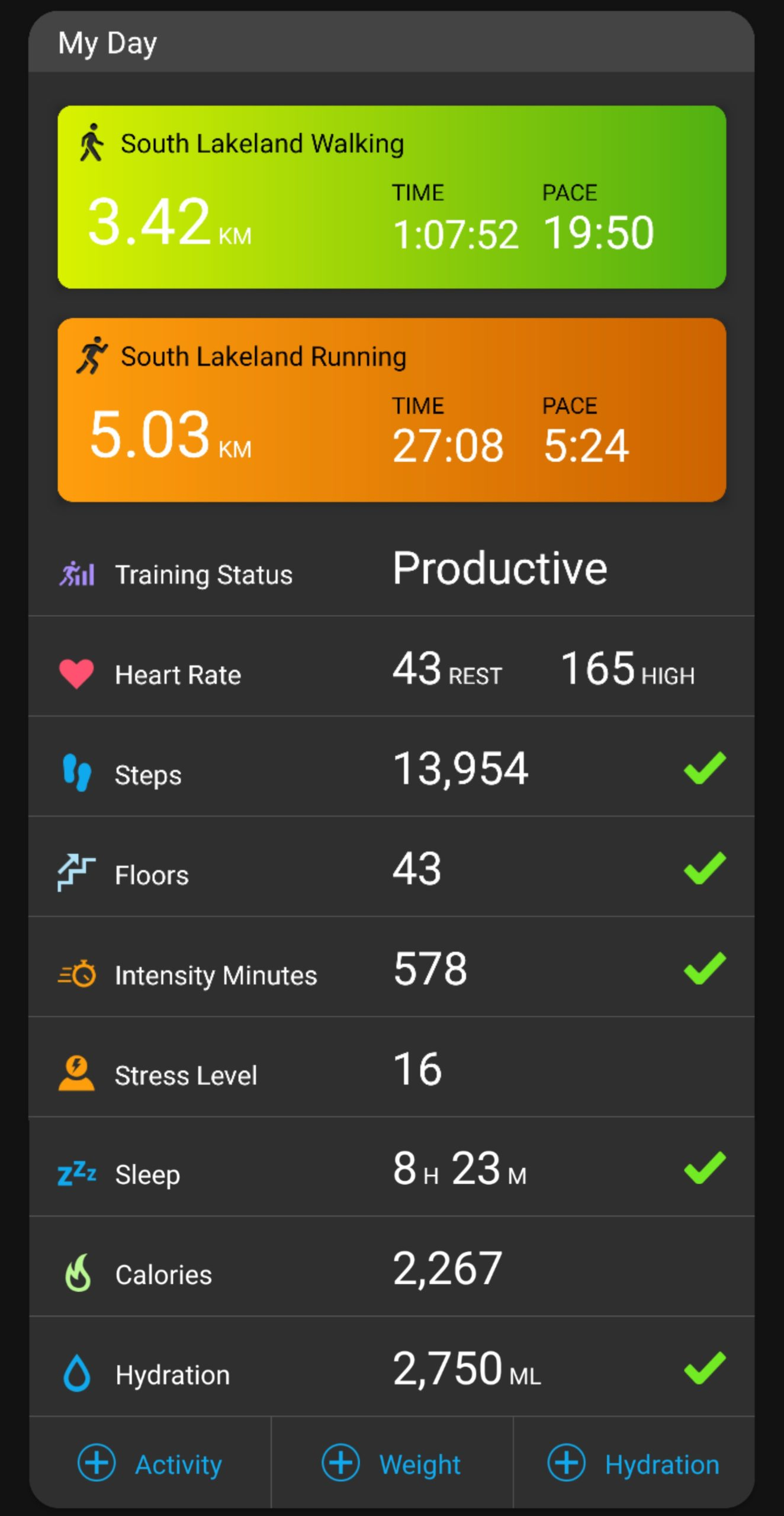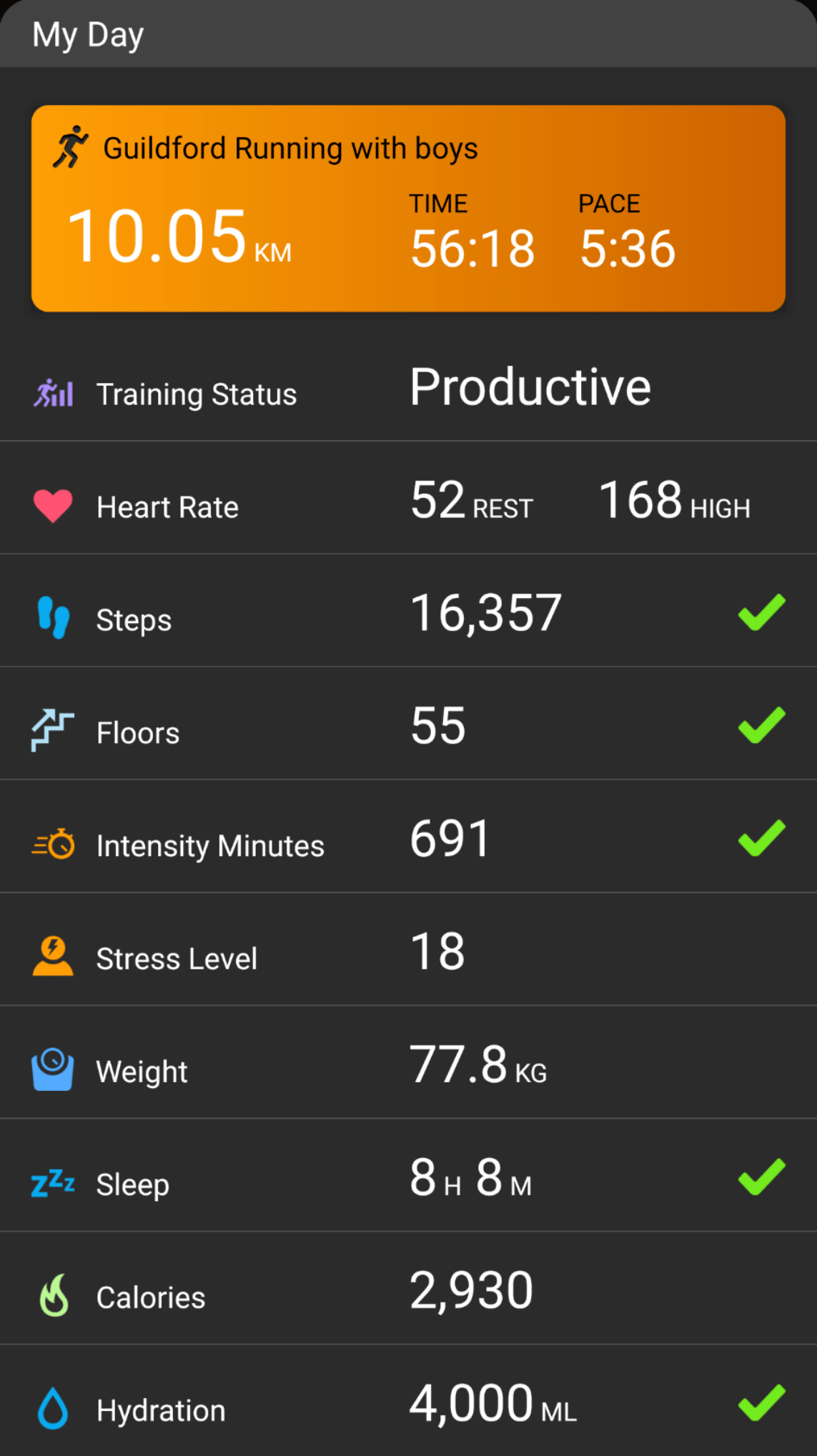## Five checkmark dayA short run around the camp, a stroll around Tarn How, and a lot of resting and eating. Happy time at happy place.

## Five checkmark day

Ran, walked the boys, went to B&Q, did some garden work, and chores around the house.

## Almost a five checkmark day

Morning in bed with coffee, books and hugging boys. Followed by another (nearly) 10K run—felt and ran better than yesterday. Then a short walk, with a little chase play, for the boys. Lazy, hot afternoon. Capped off with another evening spent in the cool river water with Chewie and Dudley. All ready to crash now. Happy! 🙂

## 5 check mark dayLast one was more than two months ago; I was more than two kilos lighter and much fitter.

Hopefully I won’t wait another 2+ months for another.

## Two 5 check mark days in a row!## Five check-mark dayA bit more discipline, and I’ll get another check mark in a few months. (Weight target: 75 Kg)

## Maths for fun on run

Doing simple maths is one of my favourite things to do on solo runs1. I try to calculate my pace based on distance and time on the watch2, or I try to calculate the time it’d take me to the finish, or most commonly, I calculate the pace I need to run to finish a race in under a certain time.

Today’s schedule had an easy 55 min run, so distance and pace weren’t very relevant. I was instead thinking whether I’ll complete 15000 steps by the time I got home.

I had about 4500 steps after the boys’ walk. I walked around the house a bit—changing, procrastinating, feeding the boy, warming up, etc. So, I could assume I would have had ~5000 steps when I started the run. Here’s a simplified version of how the calculation went:

My metronome was set at 182 beats per minute, so I could assume I’d have an average cadence of at least 182 steps per minute. Total steps would thus be…

= 182 * 55
= (180 + 2) * 55
= 180 * 55 + 2 * 55
= 180 * 55 + 110
= 180 * (1.1 * 50) + 110
= (180 * 1.1) * 50 + 110
= 198 * 50 + 110
= 198 * (100 / 2) + 110
= (198 / 2) * 100 + 110
= 99 * 100 + 110
= 9900 + 110
= 10010 steps

That should get me past the 15K mark :)

Later, on the way back up the hill, the watch buzzed to tell me I’d completed 10000 steps (for the day). I’d run ~27 mins at that point. It was the cue for the next mental maths for fun. Here’s how it went (again, grossly simplified):

Starting with total run time of 55 mins, steps I expected to get in the remainder of the run were:

= (55 – 27) * 182
= 28 * 182
= 28 * (180 + 2)
= 28 * 180 + 28 * 2
= 28 * 180 + 56
= (30 – 2) * 180 +56
= 30 * 180 – 2 * 180 +56
= 30 * 180 – 360 + 56
= 30 * 180 – 304
= 3 * 18 * 100 – 304
= 54 * 100 – 304
= 5400 – 304
= 5096 steps

Still on track to make it to 15000 steps.

Here’s what the watch said when I got home :)## Into year two…Step target streak completed a year yesterday.

## Answer: One hour of snoozing

Question: What happens when I leave the phone on the bed at night?